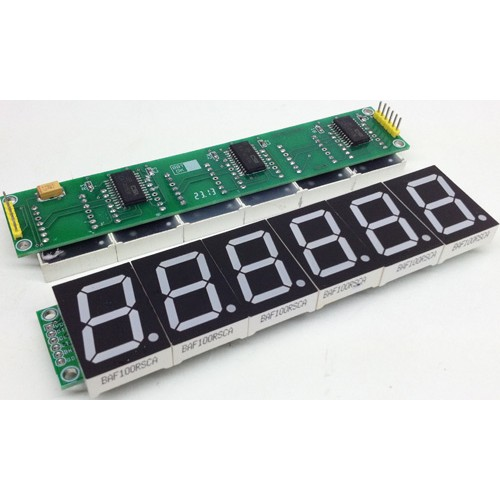# Digital Ohmmeter circuit using pic microcontroller

Digial ohmmeter using pic microcontroller is designed in this project. Digital ohmmeter is used to measure resistance of any resistance or any circuit. It can measure resistance between 0-1MΩ with precision of ±5  percent.  PIC16F877A microcontroller is used in this project to measure resistance by doing signal conditioning of measured resistance. LCD is used to display resistance value. Voltage divider concept is also in this project. I will discuss it in later part of this article.### Analog and digital ohmmeter

Analog ohm meter use electronics concepts to measure resistance and displays it on analog meters with the help of needles. Digital ohmmeter use numerical display to display resistance value like seven segment display. liquid crystal display and LED displays. Digital ohmmeter also use digital electronics to measure resistance with the help of microcomputers.

### digital ohmmeter Circuit diagram  using pic microcontroller

Circuit diagram of digital ohmmeter using pic microcontroller is given below. As shown in figure below, resistance R1 is pulled with 5 volt source and resistance whose value we want to measure is connect with ground and other terminal of resistance R1. Voltage divider concept is used to measure resistance value. LCD is used to display resistance value.### Digital ohmmeter working and operation

In above circuit diagram resistance R1 is used to as pull up resistor. Resistance R1 is connected to 5 volt source. The resistance whose value we want to measure is connected with resistance R1 to form a voltage divider. I have already discussed in Digital voltmeter article about how to measure voltage using pic microcontroller. I also used voltage divider in digital voltmeter article to design digital voltmeter. Same concept is used in this article. you should also know about LCD interfacing with pic microcontroller. If you don’t know about voltage measurement and lcd interfacing, I recommend you to read following articles first:

READ  My GPS LCD Display Project using PIC16F84

For more detail: Digital Ohmmeter circuit using pic microcontroller

#### Current Project / Post can also be found using:

• microhcip digital ohmmeter example
• suppliers of ohmmeter contact us mail